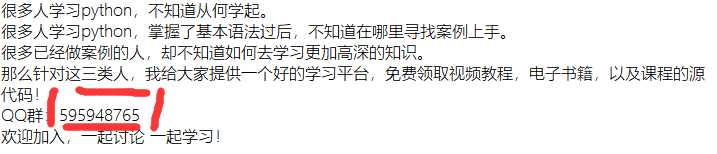# 短线买股赚钱的概率有多大？python带你来分析

### 短线交易模型的由来A 股大多数交易者都喜欢短线交易，他们都希望今天买入，明天就卖出，以此来赚快钱，殊不知短线交易是大多数人亏损最快的方式。

A 股市场上有一批最顶尖的高手，他们可以做到八年一万倍的神话，这类高手惊人收益率的来源就是短线交易，他们的镰刀专门伸向追涨杀跌的韭菜。1. 先将买入的营业部买入净额排序，换算成比例，例如：买入1:买入2:买入3:买入4:买入5=ac:d:e；
2. 查看营业部上榜后的上涨概率，例如为：A,B,C,D,E；
3. 查看营业部上榜后的平均涨幅，例如为：A1,B1,C1,D1,E1；
4. 计算综合上涨概率，公式为：(a A+b B+c C+d D+e*E)/(a+b+c+d+e)
5. 计算综合涨幅，公式为：(a A1+b B1+c C1+d D1+e*E1)/(a+b+c+d+e)
6. 计算得到的概率值越大越好，越大越值得搏击

### 数据准备

#### 龙虎榜数据```http://data.eastmoney.com/DataCenter_V3/stock2016/TradeDetail/pagesize=50,page=1,sortRule=-1,sortType=,startDate=2020-03-20,endDate=2020-03-23,gpfw=0,js=var%20data_tab_2.html?rt=26416119
```

```def parse_data(self, html, lhb_date, scode):
all_content = re.findall(pattern_all, html)
#print(all_content)

obj_list=[]
if len(all_content):
sell_content = str(all_content)

sell_objs = self.parse_detail(sell_content, 1, lhb_date, scode)
obj_list.extend(sell_objs)

return obj_list

def parse_detail(self, content, type, lhb_date, scode):
pattern = re.compile(‘[\s\S]*?<tr>[\s\S]*?<div class="sc-name">[\s\S]*?<a href=""><a href="/stock/([\s\S]*?).html">([\s\S]*?)</a></a>[\s\S]*?<div class="times-percent[\s\S]*?<div class="left">[\s\S]*?<span class="times">([\s\S]*?)</span><span[\s\S]*?>([\s\S]*?)</span>[\s\S]*?<div class="right">[\s\S]*?<td[\s\S]*?>([\s\S]*?)</td>[\s\S]*?<td>([\s\S]*?)</td>[\s\S]*?<td[\s\S]*?>([\s\S]*?)</td>[\s\S]*?<td[\s\S]*?>([\s\S]*?)</td>[\s\S]*?<td[\s\S]*?>([\s\S]*?)</td>[\s\S]*?</tr>[\s\S]*?‘)
items = re.findall(pattern, content)
obj_list=[]
for item in items:
obj = {}
obj[‘sales_code‘] = self.clear_quote(item) #营业部code
obj[‘sales_name‘] = self.clear_quote(item) #营业部名称
obj[‘his_rank_times‘] = NumUtils.trans_int(self.clear_quote(item)) #历史上榜次数
obj[‘his_rank_rate‘] = NumUtils.trans_float(self.clear_quote(item)) #历史买入3天上涨概率
obj[‘sell_money‘] = NumUtils.trans_float(self.clear_quote(item)) #卖出额
obj[‘sell_of_total_rate‘] = NumUtils.trans_float(self.clear_quote(item)) #卖出额占成交额比例
obj[‘net_money‘] = NumUtils.trans_float(self.clear_quote(item)) #净买入额
obj[‘type‘] = type #买卖类型，0-买入，1-卖出
obj[‘tdate‘] = lhb_date
obj[‘scode‘] = scode
obj_list.append(obj)

return obj_list```

#### 个股详情数据pn：页码

pz：每页记录数

fields：个股详情字段

### 回溯验证

#### 每日选股

```def ana(self, detail_list):
scode_dict = {}
rank_list = []
for detail in detail_list:
if detail[‘scode‘] not in scode_dict.keys():
sub_list = [detail]
scode_dict[detail[‘scode‘]] = sub_list
else:
sub_list = scode_dict[detail[‘scode‘]]
sub_list.append(detail)
scode_dict[detail[‘scode‘]] = sub_list

for key in scode_dict.keys():
scode = key
sub_list = scode_dict[key]
# 总买入额
total_money = float(0)
# 营业部上涨概率
yyb_avg_rate_list = []
for detail in sub_list:
yyb_avg_rate_list.append(float(detail[‘his_rank_rate‘]))

# 买入额占比
money_rate_list = [float(item[‘buy_money‘])/total_money for item in sub_list]
total_rate = float(0)
for i in range(0, len(sub_list)):
total_rate = float(total_rate) + float(yyb_avg_rate_list[i] * money_rate_list[i])
rank_list.append({‘scode‘: scode, ‘total_rate‘: total_rate})

rank_list.sort(key=lambda it: it.get(‘total_rate‘), reverse=True)
return rank_list```

#### 计算选股的上涨幅度

```def compute(self, date, stat_type):
### stat_type 表示统计类型，1-计算两天，2-计算三天 ###
# 1. 获取每日的龙虎榜选股(根据概率倒序)
lhbpick = lhb_history_pick.lhbHisPick()
pick_list = lhbpick.deal(date)

if not len(pick_list):
return None

# 2. 根据选股计算上涨点数
for stock in pick_list:
# 将退市警示股和新股排除
if ‘ST‘ in stock[‘name‘] or ‘*ST‘ in stock[‘name‘] or ‘N‘ in stock[‘name‘]:
continue

# 获取股票三日行情数据
stock_day_detail = self.query_stock_detail(stock[‘scode‘], date)
# 选股当天收盘价
day_close_price = stock_day_detail[‘close_price‘]
# 选股后一天涨停价
first_day_limit = stock_utils.StockUtils.calc_limit_price(day_close_price)
# 选股后一天最低价
first_day_low_price = stock_day_detail[‘low_price‘]
# 选股后一天最高价
first_day_top_price = stock_day_detail[‘top_price‘]
# 选股后一天开盘价
first_day_open = stock_day_detail[‘open_price‘]
# 选股后一天平均价
first_day_avg_price = (first_day_top_price + first_day_low_price) / 2
# 开盘即涨停并且一天未开板，买不进，放弃
if first_day_low_price == first_day_top_price or first_day_limit == first_day_open:
continue

# 选股后二天最低价
second_day_low_price = stock_day_detail[‘low_price‘]
# 选股后二天最高价
second_day_top_price = stock_day_detail[‘top_price‘]
# 选股后二天平均价
second_day_avg_price = (second_day_top_price + second_day_low_price) / 2

# 计算上榜后两天的情况
optim_up2 = (second_day_top_price - first_day_low_price) / first_day_low_price
pessim_up2 = (second_day_low_price - first_day_top_price) / first_day_top_price
avg_up2 = (second_day_avg_price - first_day_avg_price) / first_day_avg_price

if stat_type == 1:
# print(optim_up2, pessim_up2, avg_up2)
return optim_up2, pessim_up2, avg_up2

# 选股后三天最低价
third_day_low_price = stock_day_detail[‘low_price‘]
# 选股后三天最高价
third_day_top_price = stock_day_detail[‘top_price‘]
# 选股后三天平均价
third_day_avg_price = (second_day_top_price + second_day_low_price) / 2

# 计算上榜后三天的情况
max2 = max(first_day_top_price, second_day_top_price)
min2 = min(first_day_low_price, second_day_low_price)
avg2 = (first_day_avg_price + second_day_avg_price) / 2

optim_up3 = (third_day_top_price - min2) / min2
pessim_up3 = (third_day_low_price - max2) / max2
avg_up3 = (third_day_avg_price - avg2) / avg2

return optim_up3, pessim_up3, avg_up3

return None```

#### 结果展示(0)
(0)